## Solutions Homework Set # 8 - Physics 122

Problem 1

Using what we learned in Physics 121, we can determine that the maximum force that can be applied to rod without causing any motion is equal to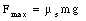The magnetic force acting on the rod is equal to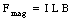We thus conclude that the maximum magnetic field for which the rod remains at rest is equal to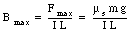Problem 2

When the current reaches a stable value (independent of time), there will be no potential drop across the solenoid, the current will be equal to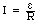The magnetic field inside the solenoid, assumed to be perfect, is equal to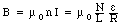Problem 3

Using the techniques we discussed in class (see equation 33.3 in the lecture notes) we can conclude that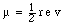Problem 4

The atomic mass of lithium is 6.941 g. The number of atoms in 6.941 g of Li is equal to NA. The magnetic moment of one Li atom is equal to 9.27 x 10-24 A m2 (see Table 33.1 in Ohanian). Assuming that all atoms in the sample are lined up, the total dipole moment of the sample is equal to the product of the number of atoms times the magnetic moment of each atom: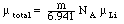The magnetic field produced by this dipole is equal to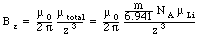Problem 5

The magnetic flux intercepted by the coil is equal towhere N is the number of turns, w and h are the width and height of the turns, B is the magnetic field, and [theta] is the angle between the normal of the coil and the magnetic field. The flux will be time dependent since the angle [theta] will be time dependent. Assuming a constant rate of rotation, we can rewrite this equation as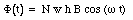The induced emf is equal to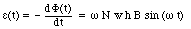The maximum emf is thus equal to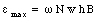The angular frequency required to achieve this emf is equal to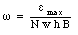Problem 6

During one complete revolution, the rod sweeps an area of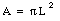Assuming the time required to complete one revolution is T, we conclude that the rate of flux change is equal to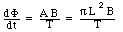The magnitude of the induced emf is thus equal to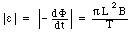Problem 7

The magnetic flux through the loop is equal to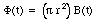The induced emf is equal to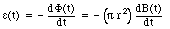The problem states that in a time interval [Delta]t the magnetic field changes from B to fB. The change in the magnetic field, [Delta]B, is thus equal to (f - 1)B. The induced emf is thus equal to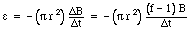Problem 8

The current induced in the loop is equal to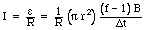Problem 9

Define t = 0 to be the time just before the loop is being pulled. The magnetic flux though the loop at this time is equal to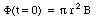The flux at time t, when the loop is pulled out of the magnetic field region, is equal to 0. Thus, the rate of the change of flux is equal to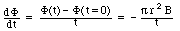The induced emf is equal to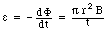Problem 10

The magnetic field generated by a current-carrying wire is equal to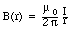Consider a small time interval dt. During this time interval the rod moves a distance v dt. The flux that is swept by the rod during this time interval is equal to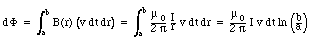The rate of flux change is thus equal to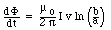The magnitude of the induced emf is equal to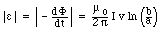Problem 11

Consider the ring at time t. The enclosed flux at this time is equal to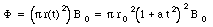The induced emf is equal to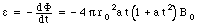The total resistance of the loop at time t is equal to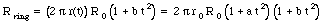The induced current is thus equal to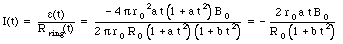Problem 12

The change in flux that is enclosed by the circuit during a time interval dt is equal to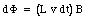where L is the distance between the rails, B is the magnetic field, and v is the velocity of the bar. The induced emf in the bar is equal to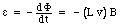The magnitude of the induced current is equal to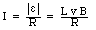The magnetic force acting on the rod as it moved through the magnetic field is equal to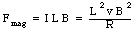Since the rod is moving with constant velocity v, the net force on the rod must be zero, and the external applied force must be equal in magnitude, but opposite in direction to the magnetic force: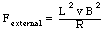Problem 13

The energy dissipated in the current loop is equal to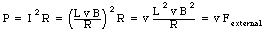We conclude that the work done by the external force is converted into Joule heating in the circuit.